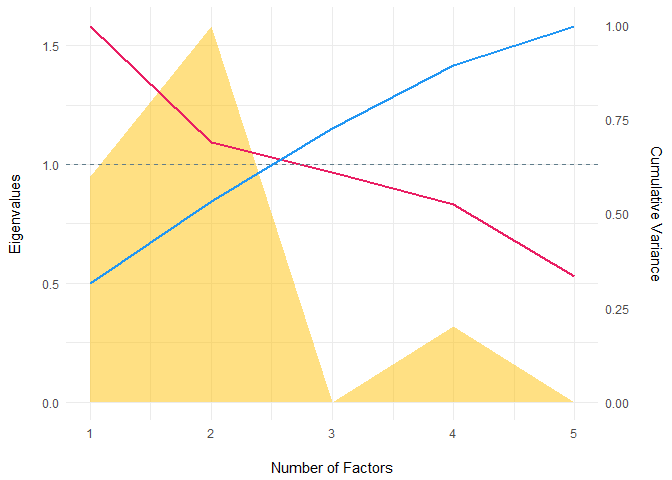Want to share your content on R-bloggers? click here if you have a blog, or here if you don't.

# The method agreement procedure

When running a factor analysis, one often needs to know how many components / latent variables to retain. Fortunately, many methods exist to statistically answer this question. Unfortunately, there is no consensus on which method to use. Therefore, the `n_factors()` function, available in the psycho package, performs the method agreement procedure: it runs all the routines and returns the number of factors with the highest consensus.

```# devtools::install_github("neuropsychology/psycho.R")  # Install the last psycho version if needed

library(tidyverse)
library(psycho)

results <- attitude %>%
psycho::n_factors()

print(results)

## The choice of 1 factor is supported by 5 (out of 9; 55.56%) methods (Optimal Coordinates, Acceleration Factor, Parallel Analysis, Velicer MAP, VSS Complexity 1).
```

We can have an overview of all values by using the `summary` method.

n.Factors n.Methods Eigenvalues Cum.Variance
1 5 3.72 0.53
2 3 1.14 0.69
3 1 0.85 0.81
4 0 0.61 0.90
5 0 0.32 0.95
6 0 0.22 0.98
7 0 0.14 1.00

And, of course, plot it 🙂

```plot(results)
```The plot shows the number of methods (in yellow), the Eigenvalues (red line) and the cumulative proportion of explained variance (blue line).

For more details, we can also extract the final result (the optimal number of factors) for each method:

Method n_optimal
Optimal Coordinates 1
Acceleration Factor 1
Parallel Analysis 1
Eigenvalues (Kaiser Criterion) 2
Velicer MAP 1
BIC 2
VSS Complexity 1 1
VSS Complexity 2 2

# Tweaking

We can also provide a correlation matrix, as well as changing the rotation and the factoring method.

```df <- psycho::affective

cor_mat <- psycho::correlation(df)
cor_mat <- cor_mat\$values\$r

results <- cor_mat %>%
psycho::n_factors(rotate = "oblimin", fm = "mle", n=nrow(df))

print(results)

## The choice of 2 factors is supported by 5 (out of 9; 55.56%) methods (Parallel Analysis, Eigenvalues (Kaiser Criterion), BIC, Sample Size Adjusted BIC, VSS Complexity 2).

plot(results)
```# Credits

This package helped you? Don’t forget to cite the various packages you used 🙂

You can cite `psycho` as follows:

• Makowski, (2018). The psycho Package: an Efficient and Publishing-Oriented Workflow for Psychological Science. Journal of Open Source Software, 3(22), 470. https://doi.org/10.21105/joss.00470